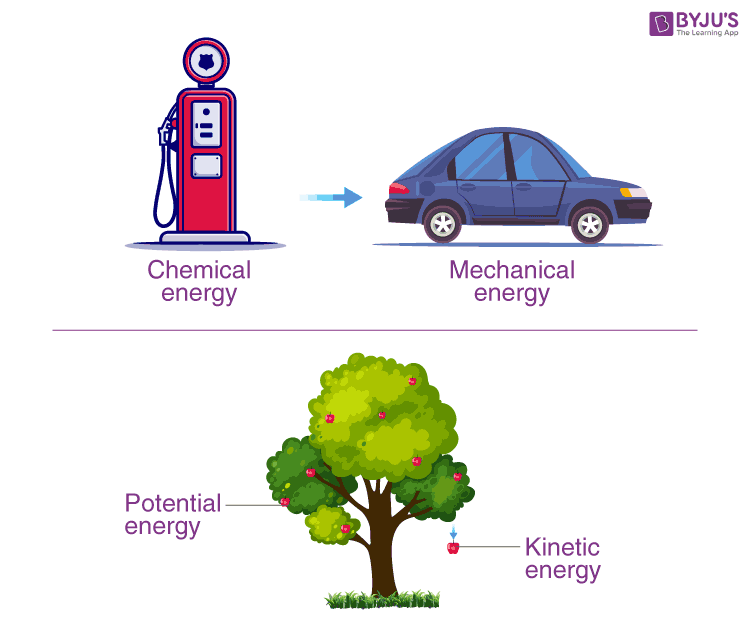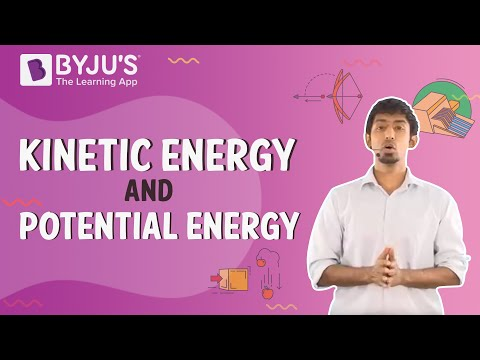# Law of Conservation of Energy

Energy is required for the evolution of life forms on earth. In physics, it is defined as the capacity to do work. We know that energy exists in different forms in nature. You have learned about various forms of energy – heat, electrical, chemical, nuclear, etc. In this article, we will learn about the laws and principles that govern energy. This law is known as the law of conservation of energy.

## What is the Law of Conservation of Energy?

The law of conservation of energy states that energy can neither be created nor be destroyed. Although, it may be transformed from one form to another. If you take all forms of energy into account, the total energy of an isolated system always remains constant. All the forms of energy follow the law of conservation of energy. In brief, the law of conservation of energy states that

In a closed system, i.e., a system that is isolated from its surroundings, the total energy of the system is conserved.Example of Energy Transformation

So in an isolated system such as the universe, if there is a loss of energy in some part of it, there must be a gain of an equal amount of energy in some other part of the universe. Although this principle cannot be proved, there is no known example of a violation of the principle of conservation of energy.

The amount of energy in any system is determined by the following equation:
UT = Ui + W + Q

• UT is the total energy of a system
• Ui is the initial energy of a system
• Q is the heat added or removed from the system
• W is the work done by or on the system

The change in the internal energy of the system is determined using the equation
ΔU = W + Q

## Law of Conservation of Energy Derivation

Considering the potential energy at the surface of the earth to be zero. Let us see an example of a fruit falling from a tree.

Consider a point A, which is at height ‘H’ from the ground on the tree, the velocity of the fruit is zero hence potential energy is maximum there.

E = mgH ———- (1)

When the fruit falls, its potential energy decreases, and kinetic energy increases.

At point B, which is near the bottom of the tree, the fruit is falling freely under gravity and is at a height X from the ground, and it has speed as it reaches point B. So, at this point, it will have both kinetic and potential energy.

E = K.E + P.E

P.E = mgX ——— (2)

According to the third equation of motion,

$$\begin{array}{l}v^{2 }= 2g(H – X)\\ \\ \Rightarrow \frac{1}{2}mv^{2}=\frac{1}{2}m.2g(H – X)\\ \\ \Rightarrow K.E=\frac{1}{2}m.2g(H – X) \\ \Rightarrow K.E=mg(H – X)\end{array}$$

K.E=mg(H-X)——– (3)

Using (1), (2) and (3)

E = mg(H – X) + mgX

E = mg(H – X + X)

E = mgH

Similarly, if we see the energy at point C, which is at the bottom of the tree, it will come out to be mgH. We can see as the fruit is falling to the bottom, here, potential energy is getting converted into kinetic energy. So there must be a point where kinetic energy becomes equal to potential energy. Suppose we need to find that height ‘x’ from the ground. We know at that point,

K.E = P.E

$$\begin{array}{l} P.E = K.E = \frac {E}{2} ——– (4) \end{array}$$

As the body is at height X from the ground,

P.E = mgX ——— (5)

Using (4) and (5) we get,

$$\begin{array}{l}mgX=\frac{mgH}{2}\\ \\ \Rightarrow X=\frac{H}{2}\end{array}$$

H/2 is referred to as the new height.

## You may want to watch the following video on potential energy and kinetic energy to understand better the principle of conservation of energy### Energy Conservation:

Energy conservation is not about limiting the use of resources which will finally run out altogether. The ideal way of conservation would be reducing demand on a limited supply and enabling that supply to begin to rebuild itself. Many times the best way of doing this is to replace the energy used with an alternative.

## Law of Conservation of Energy Examples:

In Physics, most of the inventions rely on the fact that energy is conserved when it is transferred from one form to another. A number of electrical and mechanical devices operate solely on the law of conservation of energy. We will discuss a few examples here.

• In a torch, the chemical energy of the batteries is converted into electrical energy, which is converted into light and heat energy.
• In hydroelectric power plants, waterfalls on the turbines from a height. This, in turn, rotates the turbines and generates electricity. Hence, the potential energy of water is converted into the kinetic energy of the turbine, which is further converted into electrical energy.
• In a loudspeaker, electrical energy is converted into sound energy.
• In a microphone, sound energy is converted into electrical energy.
• In a generator, mechanical energy is converted into electrical energy.
• When fuels are burnt, chemical energy is converted into heat and light energy.
• Chemical energy from food is converted to thermal energy when it is broken down in the body and is used to keep it warm.

## The below video provides Work and Energy quiz## Frequently Asked Questions – FAQs

Q1

### What is energy?

Energy is the ability to do work.

Q2

### State the law of conservation of energy?

In a closed system, i.e., a system that is isolated from its surroundings, the total energy of the system is conserved.

Q3

### Which types of energy can be seen when a block slides down a slope?

In this phenomenon, potential energy is converted into kinetic energy.
Q4

### Give an example where potential energy is converted into kinetic energy?

When the dynamite explodes, potential energy is converted into kinetic energy.
Q5

### Give an example to prove energy is conserved?

A moving car proves that potential energy is converted into kinetic energy.

## Watch the video and solve complete exemplar questions in the chapter Work and Energy Class 9Stay tuned with BYJU’S to learn more about the law of conservation of energy, heat energy, and much more.

Test your knowledge on Law of conservation of energy